### A Brief Guide to Engineering Financial Calculations: Inflation

Inflation is a quantitative measure of how much the purchasing power of money decreases as time moves forward. (Remember that a dollar tomorrow will buy less than it does today.) Inflation is a negative compound interest, i.e., 3% inflation is like a -3% interest rate. A long period of inflation occurs in a country when the money supply (the amount of a currency that is in circulation) grows at a faster rate than the country’s rate of economic growth.

In reality, the inflation rate varies continually over time and in different regions. In this course, the inflation rate is assumed to be fixed unless stated otherwise.

Calculation:

Real Interest Rate

The real interest rate for a period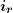is only approximately equal to the nominal inflation rate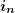minus the inflation rate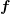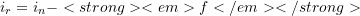The more accurate formula for real interest rate is: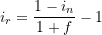If the interest rate (or discount rate) needs to include inflation, then the real interest rate should be used.

Net Present Worth including Inflation

A calculation of NPV is typically done using a nominal MARR. If inflationis a factor, then NPV should be calculated using inflation-corrected future values. This is done as follows:

• Adjust the future values in each period for inflation(the future values will go up, reflecting the equivalence associated with the declining value of money).
• Then calculate the PVs using these inflation-corrected future values and the nominal MARR. In the case of taxable income, do the inflation calculation first to get the inflation-corrected FV of the cash flows before doing the tax calculation.

An alternative approach is to use the nominal future values and a MARR that is corrected for inflation:

• Use the future values as quoted, without any inflation correction, and then use the real MARRreal = (1 + MARR)/(1 + f) – 1.

Caution: This alternative method only works if there is no intermediate calculation to do, such as taxes, and so it is prone to error. For this reason, the first method is preferred.